SOLSTICE: An Electronic Journal of Geography and Mathematics. (Major articles are refereed; full electronic archives available). Persistent URL:  http://deepblue.lib.umich.edu/handle/2027.42/58219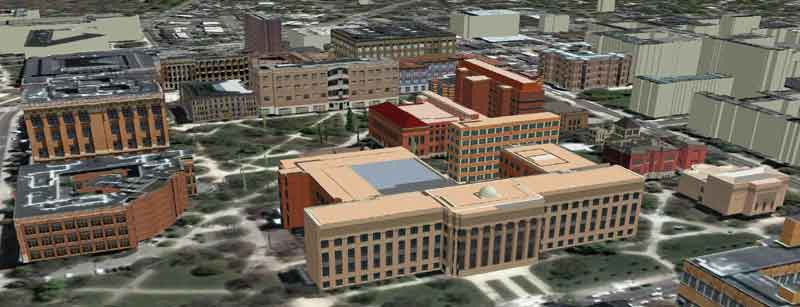Best viewed in Firefox browser (but should work in any current browser).  Works best with a high speed internet connection.
Final version of IMaGe logo created by Allen K. Philbrick from original artwork from the Founder.Educational Research Collaboration
Part 2
Sandra L. Arlinghaus, Ph.D. and Joseph J. Kerski, Ph.D.

"I believe that man will not merely endure, he will prevail."
William Faulker, Nobel Prize Acceptance Speech, December 10, 1950.
Introduction

As exciting new vistas open doors in both geographical and mathematical visualization, it may be easy to get caught up in the focus within one discipline or the other.  The richness that comes from interplay between disciplines can get cast aside, only to be rediscovered much later when single disciplines have matured and are looking for the fresh and new, beyond original disciplinary boundaries.

When such loss occurs at the research level, it is disappointing because much creative activity can slow down while more conventional activity plays out its course within established, comfortable, and conventional limits.  When such loss occurs in the education of children it can be far more dramatic, indeed tragic, as generations of future citizens, voters, municpal authorities, students, researchers, and teachers may become trapped in curricular conventions of a particular decade.

A number of scholars, in wide-ranging fields, have long seen this difficulty.  It becomes perhaps more pronounced now with the technological revolution within which so many projects, scholarly and educational alike, are set.  One form such entrapment may take is in the confusion of toolkits with concepts.  Duane Marble recently characterized this difficulty quite clearly:

"Confusing science with the tools of science is an increasing problem in our discipline. Geographic science forms the basis for spatial and spatiotemporal analysis. Over the past few decades we have developed a complex tool kit that we refer to as GIS. The tool kit exists for two reasons: the advancement of geographic science and to effectively apply many of the concepts of geographic science to the solution of a large number of the problems of our society. You cannot make effective use of GIS tools unless you understand geography.

There is also some confusion in the words that we use to talk about GIS. "Geospatial" is a term that was created by people outside of Geography who were uncomfortable with the "Geographic" part of Geographic Information Systems. "Geomatic" is a Canadian term that was set forth as a compromise term between the geography/cartography and surveying communities of that nation. It also fits well into their English/French language situation.

GIS tools have been highly successful and have opened spatial and spatiotemporal doors in many areas, both scientific and practical, that had been ignoring spatial and spatiotemporal factors since they were too difficult to deal with using the traditional, analog tool kit that we lived with for so long."

We have both been interested, for at least a part of our careers, in helping to bridge the interdisciplinary gap between geography and mathematics (particularly geometry).    One reflection of our interest appears in a previous article (the first in the MatheMaPics set) while another appears in a forthcoming book (Spatial Mathematics:  Theory and Practice through Mapping).

Reflections

In the first article in this series, we focused on "finding" things:  finding "size," finding the "center," and finding a "path."  All of these are broad concepts; all have a spatial component; all have a mathematical component.  They are not part of any self-contained toolkit.  They are, rather, broad, enduring concepts that underlie a wide variety of toolkits.

The so-called "five themes" for geography of "location," "place," "human/environment interaction," "movement," and "regions" offer one way to partition conceptual material.  Since they were first announced in 1984 (Guidelines for Geographic Education, Elementary and Secondary Schools) they have served as a set of basic concepts guiding most pre-collegiate education in the United States.  While this particular partition of conceptual material is useful, an infinite number of such partitioning procedures might, however, be created--some of greater utility than others.   We consider, in this collaborative, one other basic concept here and invite colleagues from around the world to join us in this quest in future papers in this series!  The infinity of partitions available speaks to the richness of the approach.

Diffusion

The concept of "diffusion" is one that transcends scientific borders.  One can find definitions for it not only in geography but also in biology, chemistry, general science, and no doubt others as well.  Generally, it involves the spreading of something more widely.  One famous case in geography involves the spreading of information about innovative bovine tuberculosis controls.  We will consider this classical case of Hä
gerstrand and then move to look at some contemporary views that employ toolkits not available to Hägerstrand.

A Classical Vision:  Hägerstrand's Simulation of the Diffusion of an Innovation

Torsten Hägerstrand, a Swedish geographer at the University of Lund, used the following technique to trace the diffusion of an innovation.

• The thing being diffused (communicated) is an idea;
• the agents of diffusion, or carriers of new information, are human beings;
• the space in which the idea is to be diffused is a region of the world.

Hägerstrand traced the diffusion process by imitating it with numbers. Such imitation, leading to prediction or forecasting of the pattern of diffusion, is called a simulation of diffusion. To follow the mechanics of this strategy, it is necessary only to understand the concepts of ordering the non-negative integers and of partitioning these numbers into disjoint sets.

The figure on the left shows the spatial distribution of the number of individuals accepting a particular innovation after one year of observation (Hägerstrand, p. 380).  The figure on the right shows a map of the same region and of the pattern of acceptors after two years--based on actual evidence. Notice that the pattern at a later time shows both spatial expansion and spatial infill (more concentrated use and greater density per unit of land area). These two latter related concepts are also enduring ones and they appear over and over again in spatial analysis---as well as in planning at municipal and other levels.

Figure 1.
 aa a A B C D E F G H I J K L M N a a a a a a a a a a a a a a a a 1 a a a a a a a a 2 a a 1 1 a a 3 a 1 a 4 a 5 1 a 5 a a 2 a 6 a 2 7 a 1 3 a 8 a 1 1 1 a 9 a a 1 a 10 a a 1 a a a a a a a a 11 a a a a a a a a a a 12 a a a a a a a a a a

Figure 1.  Initial distribution of adopters.

Figure 2.
 aa a A B C D E F G H I J K L M N a a a a a a a a a a a a a a a a 1 a a a a a a a a 2 1 1 a a 3 a 1 1 1 a 4 a 6 1 1 1 a 5 a a 2 1 a 6 a 5 7 a 1 1 1 3 a 8 a 1 1 2 2 a 9 a a 1 1 a 10 a a 1 a a a a a a a a 11 a a a a a a a a a a 12 a a a a a a a a aa a
Figure 2.
Figure 2.  Actual distribution of adopters after two years.

Might it have been possible to make an educated guess, from Figure 1 alone, as to how the news of the innovation would spread? Or might one develop Figure 2 from the Figure 1 using some replicable, systematic process?   What are the intellectual and deep challenges to modelling such process?

• The numerical pattern displayed in the figures is discrete in nature, yet the concept it represents is a continuous one.  Thus, one is faced with the challenge of devising a strategy that satisties both the discrete character of human nature (humans are individual points) and the continous character of a flow (of information or other).
• Most of mathematics, itself, is based on the Law of the Excluded Middle (statements are 'right' or 'wrong'; 'true' or 'false'; 'black' or 'white')--it is a two-valued logic system (Blass and Harary).  There is no 'gray' area.  Yet in much of the natural world there is no 'black' or 'white'--only gray.  Thus, one is also faced with the challenge of aligning mathematical and human process and in circumscribing regions in which the model is valid.

The steps below will use the grid in Figure 3 to assign random numbers to the grid in Figure 1, producing Figure 4 as a simulated distribution, as opposed to the actual distribution of Figure 2, of acceptors after two years.

Construct a "floating" grid (Figure 3) to be placed over the grid on the map of Figure 1, with grid cells scaled suitably so that they match. Center the floating grid on a square in Figure 1 in which there exists an adopter/numeral.  The animation will show the direction of movement of the grid.

The numbers in the floating grid, used with a set of four digit random numbers, will be used to determine likely location of new adopters. It is shown in enlarged form on the right of Figure 3.   Notice that cells close to the center of the grid contain a wider interval of four digit numbers than do those near the edge.  Hence, it is more likely that a random number will fall within in an interval near the center than near the edge.  This numerical pattern reflects the idea that an individual is more likely to communicate with someone nearby than with someone far away (Tobler's Law); velocity of diffusion is expressed in terms of probability of contact.   That is, the assignment of four digit numbers reflects the probability of contact; in this case that assignment is symmetric, but asymmetric assignment might reflect decisions about boundaries and other physical or human features.  In Figure 3, there are 22 initial adopters and thus 22 random numbers.  Different sets of random numbers produce results that are different from each other in terms of detail of distribution but probably not in terms of general pattern of clustering, infill, and spatial extension.   This assumption regarding distance and probability of contact is reflected in the assignment of numerals within the grid--there are the most four digit numbers in the central cell, and the fewest in the corners. The floating grid partitions the set of four digit numbers {0000, 0001, 0002, ..., 9998, 9999} into 25 mutually disjoint subsets.  The grid together with the sets of numbers is referred to (by H
ägerstrand and others) as the Mean Information Field (MIF).6248
0925
4997
9024
7754

7617
2854
2077
9262
2841

9904
9647
3432
3627
3467

3197
6620
0149
4436
0389

0703
2105
 0000 to 0095 0096 to 0235 0236 to 0403 0404 to 0543 0544 to 0639 0640 to 0779 0780 to 1080 1081 to 1627 1628 to 1928 1929 to 2068 2069 to 2236 2237 to 2783 2784 to 7214 7215 to 7761 7762 to 7929 7930 to 8069 8070 to 8370 8371 to 8917 8918 to 9218 9219 to 9358 9359 to 9454 9455 to 9594 9595 to 9762 9763 to 9902 9903 to 9999

Figure 3.   The left shows Figure 1 with the grid on the right scaled to have its squares fit the grid on the left.  Animation shows the direction of movement in application of the grid of numbers.  Notice that the grid floats across the distribution from Figure 1 so that it is centered, from left to right, on each non-zero entry.  The middle column shows a set of random numbers taken from a table of random numbers (where one chooses them in the order presented in the table in order to preserve randomization).Figure 4.  Here the Mean Information Field collects new adopters (red dots) and inserts them in the base configuration from Figure 1.  The transformation described above is animated to illustrate it.

Figure 4 demonstrates the detail of using the Mean Information Field to generate a simulation of the diffusion of an innovation.  Center the floating grid on F2.  The first random number (from Figure 3) is 6248 and it lies in the center square of the MIF. So in the simulation, the acceptor in F2 finds another acceptor nearby in F2. Record that simulated acceptor as a red dot. Together with the original adopter, there are now two adopters in this cell.  Move the MIF over and center it on the next cell with a numeral/adopter (as suggested in the animation in Figure 3) and repeat the procedure using the next random number in the sequence.  The animation of Figure 4 shows the use of the entire set of random numbers in Figure 3 and the consequent simulated pattern of new adopters.  Figure 5 shows a static view and illustrates issues associated with edge effect problems.a a A B C D E F G H I J K L M N a a a a a a a a a a a a a a a a 1 a a a a a 1 a a a 2 a a 1+1 1 a a 3 a 1+1 a 4 a 1 5+1 1+1 1 a 5 a a 2+1 1 1 6 a 1 2+1 1 7 a 1+1 3+1+3 a 8 a 1+1 1+1+1 1 a 9 a a 1 a 10 a 1 1 a a a a a a a a 11 a a a a a a a a a a 12 a a a a a a a a a a
Figure 5.  Simulated distribution of acceptors, using random numbers.  Original acceptors in black; simulated acceptors in red.  Consider what to do with edge effect issues.  How does the simulation compare to the actual distribution of adopters after two years (Figure 2)?  This question leads to a whole set of issues about how to compare pattern--one might use color, contours, a variety of numerical measures, and so forth.

While the spatial pattern simulated is discrete, it is based on underlying continuous mathematics.  The construction of the MIF assumes, based on empirical evidence, that the frequency of social contact (communication, migration) per square kilometer falls off or decays with distance.   And, this idea is captured easily with a distance decay curve, a continuous curve, with units on the x-axis expressed in terms of kilometers and on the y-axis in terms of the number of migrating households per square kilometer.  More detailed information on the mechanics of construction is available at a variety of locations including at the following linked site

Contemporary Vision

Two toolkits that were not available to Hägerstrand for his analysis of the diffusion of an innovation were fractal geometry and Geographic Information Systems (GIS) software.  We consider superimposing these two tools on the classical idea.

A Space-Filling Vision of Diffusion

Given a view of a gridded locale in the style of Hägerstrand (Figure 6), along with an associated 5-by-5 MIF (Figure 7)  with numerical entries expressed as percentages of the numbers from Figure 3.  Thus, for example, the central cell contains 44.31 percent of the four digit numbers and the likelihood that an individual in that cell will contact another within that cell is 44.31 percent.  Probabilities of the likelihood of contact simulate the spread of knowledge of the innovation over time.  Over time, the information will spread, gaps will fill in and, eventually, the population will become saturated with the information.  Figure 6 shows a very simple pattern of initial adopters, coded as '1' in three cells:  H3, H4, and H5.  The first generation of adopters adds three more adopters, one each in cells H3, G3, and H5, using the first three random numbers from Figure 3:  H3 goes to H3 from 6248; H4 goes to G3 from 0925; and, H5 goes to H5 from 4997.  Because of the weighting of the assignment of probabilities, clearly different initial distributions of adopters will lead to different outcomes of specific locations, even though there may be general pattern similarities.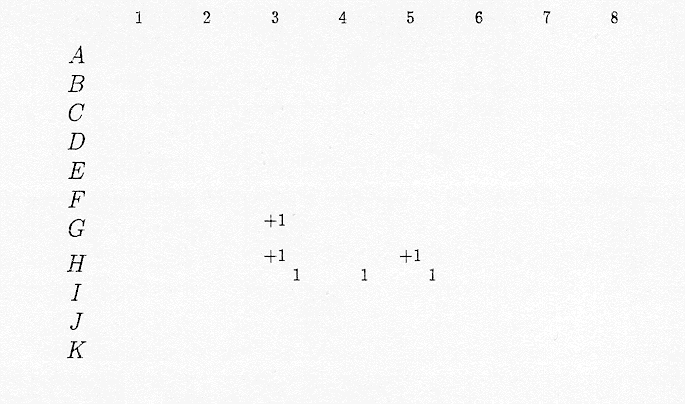Figure 6.  Initial adopters are coded 1, in cells H3, H4, and H5.  First generation adopters are coded +1, in cells H3, G3, and H5.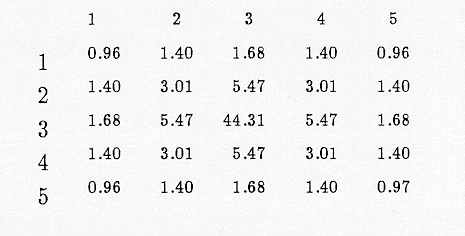Figure 7.  Mean Informtaion Field from Figure 3 with percentages of four digit numbers entered.  These percentages represent probability of contact.  Thus, when the MIF is centered on adopter, there is a 44.31% chance that his next contact will be in the same cell he is in; there is a 0.96% chance that it will be in two cells away on the diagonal, and so forth in between.
<>
Independent of how many generations are calculated using this procedure, the pattern of "filling in" of new adopters is heavily influenced by the shape of the set of original adopters.  Indeed, over time, knowledge of the innovation diffuses slowly initially, picks up in speed of transmission, tapers off, and eventually the population becomes saturated with the knowledge.  Typically, this process is characterterized as a continuous phenomenon using a differential equation of inhibited growth that has as an initial supposition that the population may not exceed M, an upper bound, and that P(t), the population P at time t, grows at a rate proportional to the size of itself and proportional to the fraction left to grow (Haggett et al., 1977; Boyce and DiPrima, 1977).  An equation such as dP(t) / dt = kP9t)(1-(P(t)/M)) serves as a mathematical model for this sort of growth in which k>0 is a growth constant and the fraction (1-(P(t)/M) acts as a damper on the rate of growth (Boyce and DiPrima, 1977).  The graph of the equation is an S-shaped (sigmoid) logistic curve with horizontal asymptote at P(t)=M and inflection point at P(t)=M/2.  When dP/dt >0 the population shows growth; when d2P/dt2 > 0 (below P(t) = M/2) the rate of growth is increasing;  when d2P/dt2 < 0 (above P(t) = M/2) the rate of growth is decreasing.  The differential equation model thus yields information concerning the rate of change of the total population and in the rate of change in growth of the total population.  It does not show how to determine M; the choice of M is given a priori.

Iteration of the Hägerstrand procedure gives a position for M once the procedure has been run for all the generations desired.  For, it is a relatively easy matter to accumulate the distributions of adopters and stack them next to each other, creating an empirical sigmoid logistic curve based on the simulation (Haggett et al., 1977).  Finding the position for the asymptote (or for an upper bound close to the asymptotic position) is then straightforward.

Neither the Hägerstrand procedure nor the inhibited growth model provides an estimate of saturation level (horizontal asymptote position) (Haggett, et al., 1977) that can be calculated in the measurement of the growth.  The fractal approach suggested below offers a means for making such a calculation when self-similar hierarchical data are involved; allometry is a special case of this procedure (Mandelbrot, 1983; Michigan Inter-University Community of Mathematical Geographers).  The reasons for wanting to make such a calculation might be to determine where to position adopter 'seeds' in order to produce various levels of innovation saturation.  The following example illustrates how a fractal/space filling approach, based on self-similarity, can offer measures, at the outset and based only on the positions of the initial adopters, of eventual saturation.

To follow the mechanics of the process, suppose, in Figure 8a, that the grid of Figure 7 is superimposed and centered on the original adopter in cell H3.  A probability of 3.01% is assigned to the likelihood for contact from H3 to G4.  When the grid is superimposed and cnetered on the original adopter in H4, there is a 5.47% likelihood for contact from H4 to G4.  And, when the grid is superimposed and centered on the original adopter in H5, there is once again a 3.01% likelihood for contact from H5 to G4.  Therefore, the percentage likelihood of a new first-generation adopter in cell G4, given this initial configuration of adopters, is the sum of the percentages dificed by the number of initial adopters, or 11.49/3.  For ease in serting fractions into a grid, only the numerator, 11.49, is shown as the entry (Figure 8a).  Then, the procedure is repeated for each cell in configuration.  It is only in zones of overlap of the grid, as it moves from one original adopter to the next that the entries in this table will differ from those in the MIF of Figure 7.  This zone of overlap, of at least two of the MIF positions, is called the zone of interaction.  In Figure 8a, the zone of interaction concides with the blue rectangle represening the MIF centered on the middle adopter.

Then, adjust the position of an original adopter.  The center adopter, in H4 will be moved, one unit toward the top in each of Figures 8b, 8c, 8d, 8e, and 8f.  The resulting configurations, row and column totals (with column totals constant and row total patterns changing), and zones of interaction are shown in the sequence of figures below.  In the last figure, the MIF of the center adopter has now moved out of the picture and no longer intersects the other two.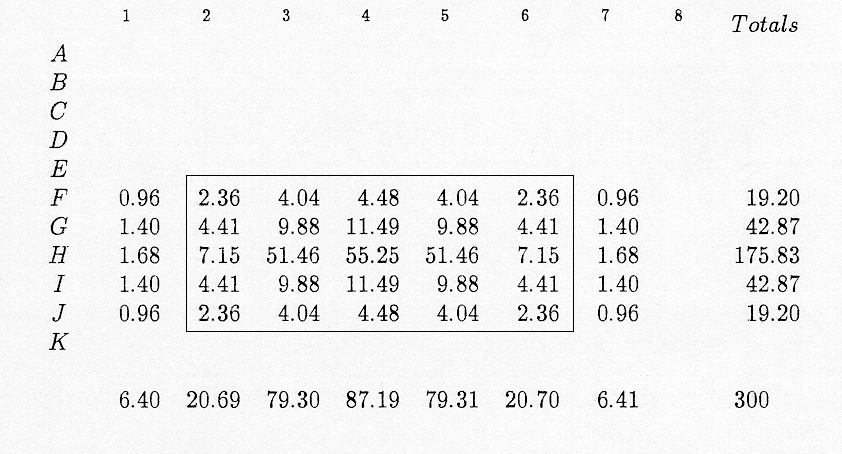Figure 8a.  Original adopters are in cells H3, H4, and H5.  The zone of interaction is composed of the intersection of any two of the three sets:  it is the blue rectangle.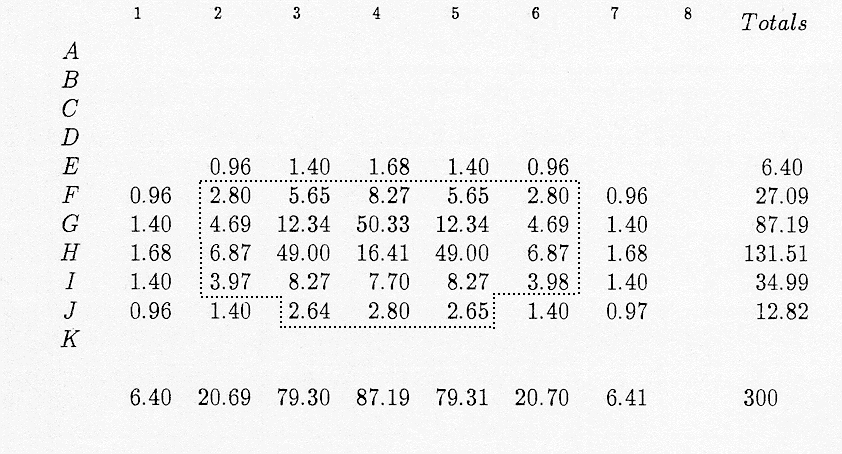Figure 8b.  Original adopters are in cells H3, G4, and H5.  The zone of interaction is composed of the intersection of any two of the three sets:  it is 'T'-shaped.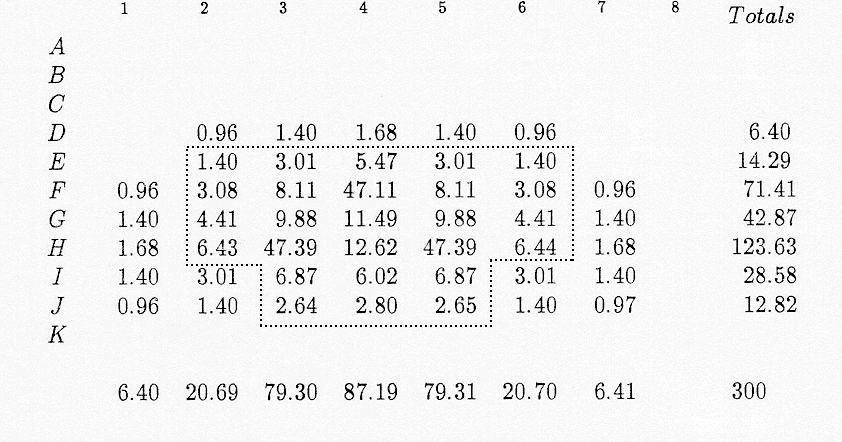Figure 8c.  Original adopters are in cells H3, F4, and H5.  The zone of interaction is composed of the intersection of any two of the three sets:  it is 'T'-shaped.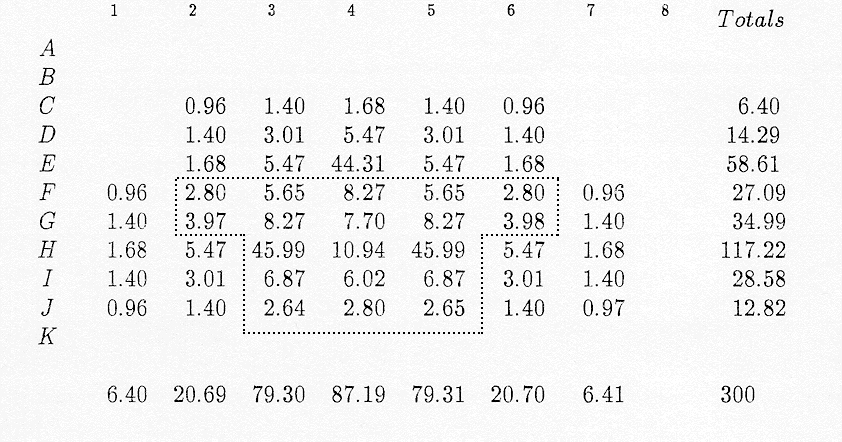Figure 8d.  Original adopters are in cells H3, E4, and H5.  The zone of interaction is composed of the intersection of any two of the three sets:  it is 'T'-shaped.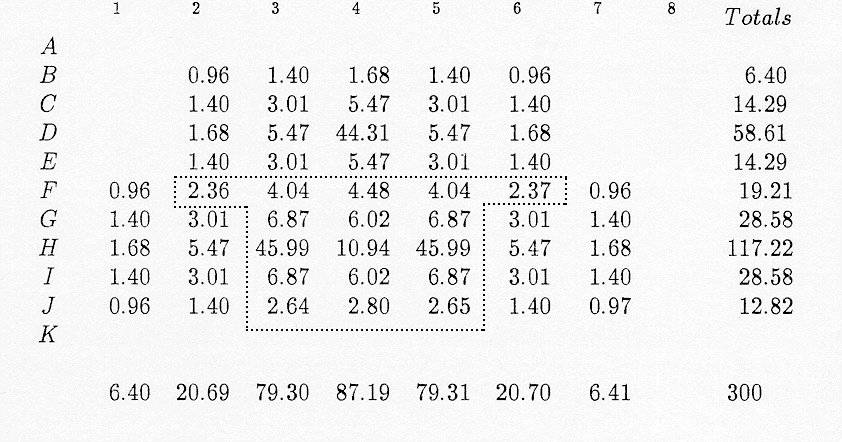Figure 8e.  Original adopters are in cells H3, D4, and H5.  The zone of interaction is composed of the intersection of any two of the three sets:  it is 'T'-shaped.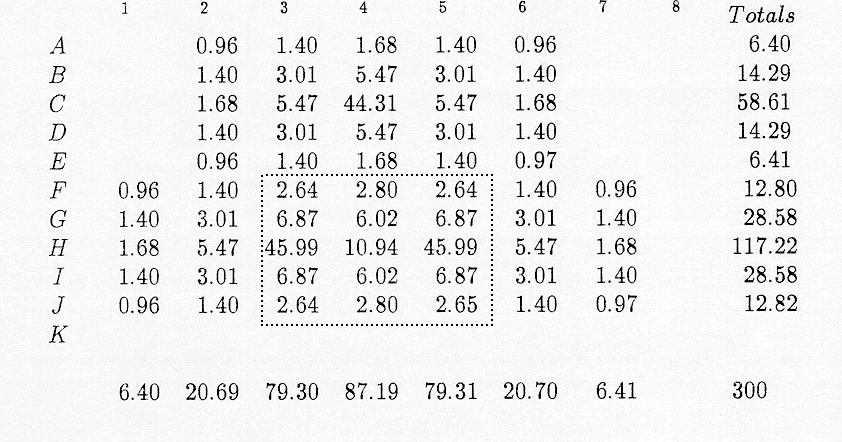Figure 8f.  Original adopters are in cells H3, C4, and H5.  Here, the zone of interaction is the red core, alone.

As the central adopter cell moves toward the top, note that naturally the associated pattern within the interaction zone preserves the same bilateral symmetry (up to truncation) as the underlying root MIF.  Thus, any configuration characterizing the pattern in this sequence of figures should also exhibit bilateral symmetry.  One such form is a tree.  In fact, if one thinks of each of the three initial adopter cells as a point centered in a cell of 1 unit side, then the three cells in Figure 8a might be represented as a linear pattern, suggested in Figure 9a, top.  In that image, view the tree on vertices H3, H4, and H5 as a generator for a self-similarity sequence (as suggested by  the arms formed in two successive stages in that figure).  In a similar manner, other trees are generated for each movement of the central original adopter, with Figure 9b derived from Figure 8b, and so forth.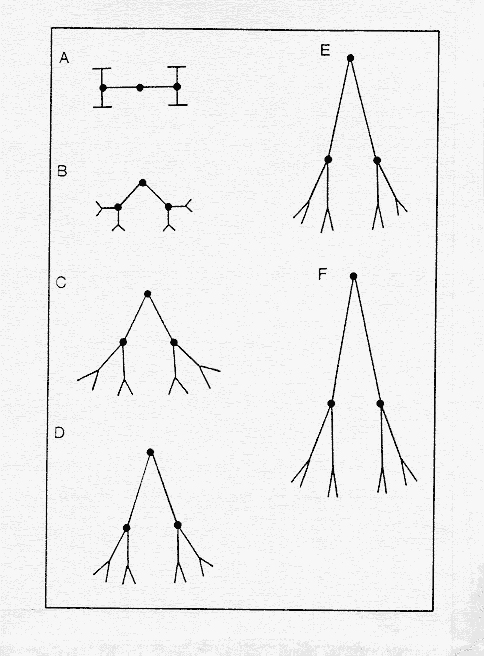Figure 9a., 9b, 9c, 9d, 9e, 9f.  Simple trees based on initial adopter entry positions, from Figures 8a, 8b, 8c, 8d, 8e, and 8f, are used to create self-similar entities as suggested above.  The red dots aid in seeing how angle theta/2 was created in each case, as the inverse tanget of 1, 1/2, 1/3, 1/4, and 1/5 respectively.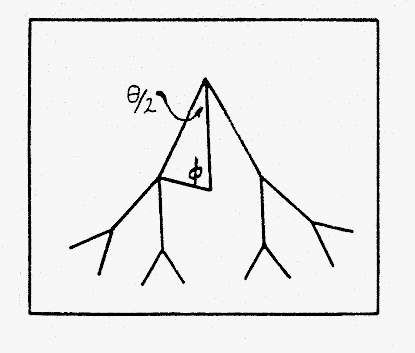Figure 10.  In this general schematic of a tree, the angle φ is created as one base angle of an isosceles triangle whose apex angle is known as θ/2 .  The length of an apical side of the triangle is determined  by  the position of the central adopter in relation to the others.

It is a straightforward matter to create the angle θ for each of the trees in Figure 9.  In Figure 9a, clearly  the angle is 180º.  In Figure 9b, it appears to be 90º but a simple calculation verifies it and sets the tone for the calculation pattern.  In Figure 9b, tan(θ/2)=1/1 so that, using the inverse tangent, θ/2 = 45º and θ=90º.

 Figure number tan (θ/2) = θ/2 = tan-1 (1/x) θ 8a 180 8b 1/1 45 90 8c 1/2 26.565... 53.13... 8d 1/3 18.435... 36.87... 8e 1/4 14.036... 28.07... 8f 1/5 11.309... 22.62...

Table 1.  Calculation of θ for each of the distributions of original adopters in Figure 8.

Certainly when looking at the geometric pattern in Figure 9, one gets an intuitive sense that Figure 8a fills the most space and Figure 8f the least, with tailing off in between.  The challenge is to find a fractal generator that will mimick the pattern, that is derived from the pattern, and will do so within the context of Mandelbrot's formula for fractal dimension, D, as D = (ln N)/(ln (1/r)) where N represents the number of sides in the generator, which in all cases here is the value 2, and where r is some sort of scaling value that remains constant independent of scale (Mandelbrot, 1983).  To find a suitable r, look back to Figure 10, and calculate
φ,  the base angle of the isosceles triangle, based on the length of the generator side.  It is constant, independent of scale.  Use the values from Table 1 for the apex angle, subtract that value from 180 and divide the result in half, to get the size of one base angle.  Table 2 shows the results of the calculation.  It also shows the value for fractional dimension using cos φ  as the value for r in Mandelbrot's formula.

 Figure number φ = (180-(θ/2))/2 D=(ln 2)/(ln (1/cos φ)) 8a 45 2 8b 67.5 0.721617... 8c 76.78 0.471288... 8d 80.78 0.378471... 8e 82.98 0.32971... 8f 84.35 0.299116...

Table 2.

Thus, a measure that reflects the degree of space-filling, based only on the pattern of original adopters,  can follow from use of fractal iteration.  It fulfills the sought purpose of being able to offer a measure of eventual saturation, at the outset of a simulation.  It is not unique and is dependent on generator formulation (as such things must be); but it adds and extra dimension to the previous analysis and it is possible because advances in technology in the latter part of the twentieth century permitted visualization of geometric form previously "seen" only through notation.

GIS software

Fractal geometry offered one great toolkit for visualization and consequent solution to new or existing problems; Geographic Information Systems software offers yet another.   The following example employs GIS software (Esri) to integrate the geographic concept of diffusion with the business concept of market competition in a spatial context.  Lists of radio stations were researched, compiled, and then mapped in ArcGIS (Figure 11).  When suitable marker symbols were introduced, it became straightforward to see the radio catchment areas for the Kansas City Royals baseball team and for the St. Louis Cardinals baseball team.  Big businesses wishing to direct the attention of baseball fans to particular products, perhaps with a regional emphasis, need look no further than toward this sort of map!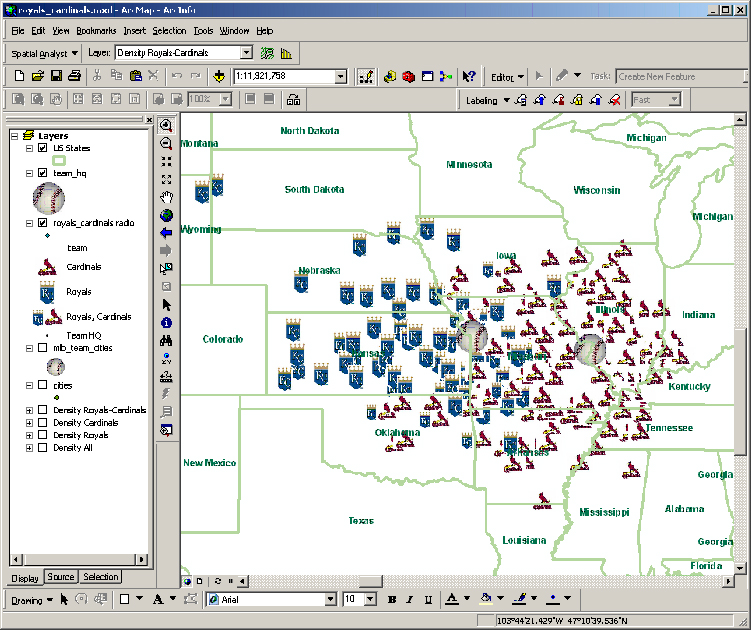Figure 11.  Radio catchment regions of St. Louis Cardinal and Kansas Royal baseball broadcasts.
These items illustrate some of our educational and scholarly interests and suggest directions for future work.  Some interactive examples are simple but not necessarily "easy"; indeed, "simple" is often the hallmark of elegance that piques curiosity and stimulates  both the motivation to learn eagerly and the imagination to pursue new directionsAs with  Faulkner's 'man', fundamental concepts such as diffusion will not merely endure,  they will prevail:  from the classical to the contemporary, in theory and in practice...all independent of associated toolkit transformations.

REFERENCES

Arlinghaus, Sandra L.  1990.  "Beyond the Fractal."  Solstice:  An Electronic Journal of Geography and Mathematics.  Volume I, No. 1.  Ann Arbor:  Institute of Mathematical Geography.  Volume also reprinted as IMaGe Monograph 13.  Both openly available at http://www.imagenet.org/ as well as in Deep Blue, the persistent electronic archive of The University of Michigan.

Arlinghaus, Sandra and Kerski, Joseph.  2010.  MatheMaPicsSolstice:  An Electronic Journal of Geography and Mathematics.  Volume XXI, No. 1.   Ann Arbor:  Institute of Mathematical Geography.   Also in Deep Blue, as above.

Arlinghaus, Sandra and Kerski, Joseph. 2013.  Spatial Mathematics:  Theory and Practice through Mapping.  Boca Raton:  CRC Press, a Division of Taylor and Francis.

Blass, A. and Harary, F.  Properties of almost all graphs and complexes.  Journal of Graph Theory 3 (1979), 225-240.

Boyce, W. E. and DiPrima, R. C.   1977.  Elementary Differential Equations.  New York:  Wiley.

Guidelines for Geographic Education, Elementary and Secondary Schools.  1984.  National Council for Geographic Education and Association of American Geographers.

Hägerstrand, Torsten.  1967.  Innovation Diffusion as a Spatial Process. Translated by Allan Pred.  Chicago:  University of Chicago Press.

Haggett , P.; Cliff, A. D.; and Frey, A.  1977.  Locational Analysis in Human Geography.  New York:  Wiley.

Kerski, Joseph.  2008.  "Baseball Radio Station Analysis."

Kerski, Joseph.  2009.  "Making Your Point with Marker Symbols."  http://www.esri.com/news/arcuser/0109/files/marker.pdf

Mandelbrot, B.  1983.  The Fractal Geometry of Nature.  New York:  W. H. Freeman.

Marble, Duane.  Association of American Geographers, April 2013.

Geospatial and Geographical analysis. An example for kids

Michigan Inter-University Community of Mathematical Geographers, series reprinted available online,  and in Deep Blue, the persistent archive of The University of Michigan.

Tobler W., (1970) "A computer movie simulating urban growth in the Detroit region".
Economic Geography, 46(2): 234-240.

 .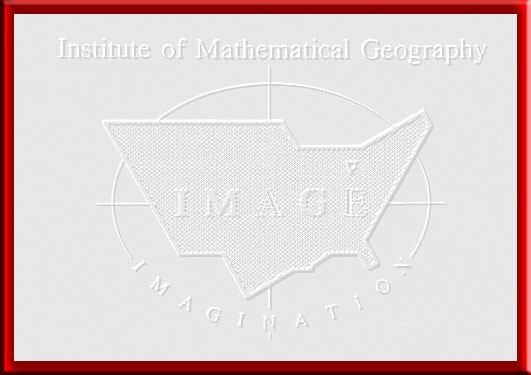Solstice:  An Electronic Journal of Geography and Mathematics,  Volume XXIV, Number 1 Institute of Mathematical Geography (IMaGe). All rights reserved worldwide, by IMaGe and by the authors. Please contact an appropriate party concerning citation of this article: sarhaus@umich.edu http://www.imagenet.org http://deepblue.lib.umich.edu/handle/2027.42/58219 Solstice was a Pirelli INTERNETional Award Semi-Finalist, 2001 (top 80 out of over 1000 entries worldwide) One article in Solstice was a Pirelli INTERNETional Award Semi-Finalist, 2003 (Spatial Synthesis Sampler). Solstice is listed in the Directory of Open Access Journals maintained by the University of Lund where it is maintained as a "searchable" journal. Solstice is listed on the journals section of the website of the American Mathematical Society, http://www.ams.org/ Solstice is listed in Geoscience e-Journals IMaGe is listed on the website of the Numerical Cartography Lab of The Ohio State University:  http://ncl.sbs.ohio-state.edu/4_homes.html Congratulations to all Solstice contributors. Remembering those who are gone now but who contributed in various ways to Solstice or to IMaGe projects, directly or indirectly, during the first 25 years of IMaGe: Allen K. Philbrick | Donald F. Lach | Frank Harary | H. S. M. Coxeter | Saunders Mac Lane | Chauncy D. Harris | Norton S. Ginsburg | Sylvia L. Thrupp | Arthur L. Loeb | George Kish |
1964 Boulder Drive,
Ann Arbor, MI 48104
734.975.0246

http://deepblue.lib.umich.edu/handle/2027.42/58219
sarhaus@umich.edu